# Beauty quarks test lepton universality

23 March 2018

A series of measurements from LHCb and B-factory experiments have tested whether the interactions of charged leptons agree with the Standard Model.The decay of a B0 meson into a K*0 and an electron–positron pair in LHCb, showing a close-up of the energy deposits of the final-state particles in the calorimeters. Image credit: LHCb

Of all the puzzling features of the Standard Model of particle physics (SM), one of the most vexing is the arrangement of the elementary particles into families or generations. Each pair of fermions comes in three and apparently only three copies: the electron, muon, tau leptons and their associated neutrinos, and three pairs of quarks. The only known difference between generations is the different strengths of their interactions with the Higgs field, known as the Yukawa couplings. This results in different masses for each particle, giving a wide range of experimental signatures.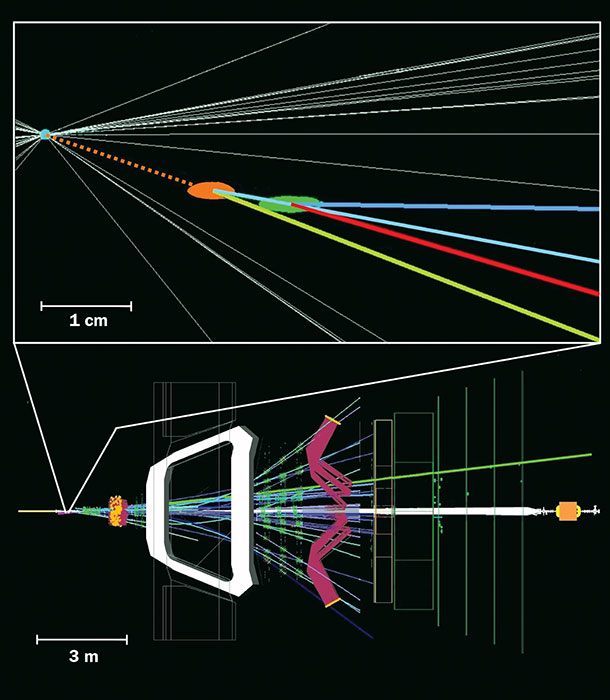Side view of an event in the LHCb detector and an enlarged area close to the interaction point, showing the decay of a B meson produced in the proton–proton interaction.

In the case of the charged leptons (electrons, muons and taus), this pattern also results in one simple post-diction, known as lepton universality (LU): other than effects related to their different masses, all the SM interactions treat the three charged leptons identically. During the past couple of decades, LU has been tested to sub-percent precision in interactions of photons and weak bosons, and in transitions between light quarks. These measurements were made, for example, at the Large Electron–Positron (LEP) collider at CERN in decays of W and Z bosons, by the PIENU and NA62 fixed-target experiments in decays of pions and kaons, and in J/ψ decays by the BES-III, CLEO and KEDR collaborations. However, LU has never been established to such a degree of precision in decays of heavy quarks.

Measurements from Run 1 of decays of beauty hadrons at the LHCb experiment, in addition to earlier results from the B-factories Belle at KEKB and BaBar at PEP-II, have hinted at potential deviations from LU. None is statistically significant on its own but, taken together, the results have led to speculation on whether non-SM forces exist or phenomena that treat leptons differently depending on their flavour are at play. If a deviation from LU was to be confirmed, it would be clear evidence for physics processes beyond the SM and perhaps a sign that we are finally moving towards an understanding of the structure of the fermions.

### Two classes

The results so far concern two classes of transitions in b-quark hadron decays, exemplified in figure 1. Measurements of highly suppressed flavour-changing neutral-current (FCNC) decays, b s+, hint at a difference involving muons and electrons, while measurements of the more frequent leading-order or tree-level decays, b c+ν, hint at a difference between muons and taus. These two classes of decays present very different challenges, both experimentally and theoretically. The latter, semi-leptonic, decays of b-quark hadrons proceed through tree-level diagrams in which a virtual W boson decays into a lepton–neutrino pair. Measurements of decays involving electrons and muons show no deviations with respect to the SM within the current level of precision. In contrast, measurements of decays involving τ leptons are only marginally in agreement with the SM expectation. The quantity that is experimentally measured is the ratio of branching fractions RD(*) = BF(B D(*)τ+ντ)/BF(B D(*)+ν), with = e or μ. This ratio is precisely predicted in the SM owing to the cancellation of the leading uncertainty that stems from the knowledge of the decay form-factors.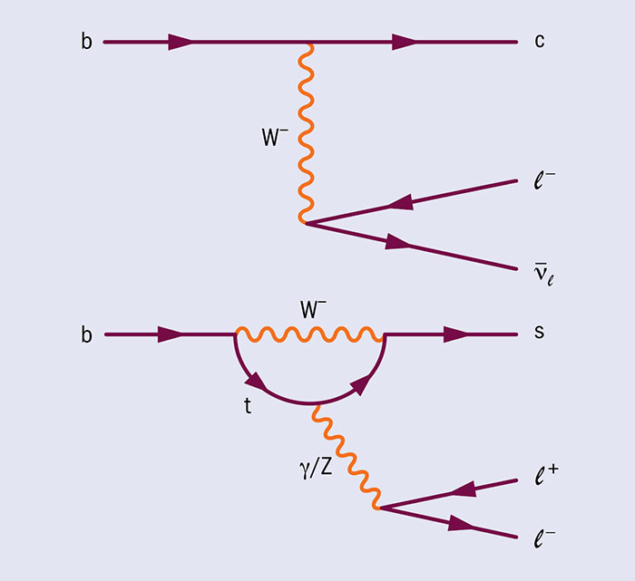Fig. 1. Feynman diagrams in the Standard Model for two classes of processes relevant to the study of lepton universality in heavy quarks: a charged-current b → cℓ–ν̅ℓ tree-level transition (top) and a neutral-current b → sℓ+ℓ− loop-level transition (bottom).

Interest in these decay modes was heightened in 2012 when the BaBar collaboration found values for RD and RD* above the SM prediction. This was followed in 2015 by results from the Belle collaboration that were also consistently high. Experimentally, such semi-tauonic beauty decays are extremely difficult to measure because taus are not reconstructed directly and at least two undetected neutrinos are present in the final state. To get around this, the BaBar and Belle experiments used both B mesons produced from Υ(4S) decays. By reconstructing the decay of one B meson in the event, the teams were able to infer the recoil of the other, “signal”, B decay. This tagging technique, based on the known momentum of the initial-state positron–electron pair and therefore that of the Υ(4S), allows the determination of the momentum of the B signal, the reconstruction of its decay under the assumption that only neutrinos escape detection, and the separation of signal and background.

The study of beauty-hadron decays to final states involving τ leptons was deemed not to be feasible at hadron colliders such as the LHC. This is a result of the unknown momentum of the colliding partons and the significantly more complex environment with respect to electron–­positron B-factories in terms of particle densities, detector occupancy, trigger and detection efficiencies. However, due to the significant Lorentz boost and the excellent performance of the LHCb vertex locator, the decay vertices of the b-hadrons produced at the LHC are well separated from the proton–proton interaction point. This enables the collaboration to approximate the b-hadron momentum and its decay kinematics with sufficient resolution to preserve the discrimination between signal and background.

### Exploiting the tau

The first measurement of RD* at a hadron collider was performed by LHCb researchers in 2015 using the decays of the τ lepton into a muon and two neutrinos. This measurement again came out higher than the SM prediction, thus strengthening the tension between theory and experiment raised by Belle and BaBar.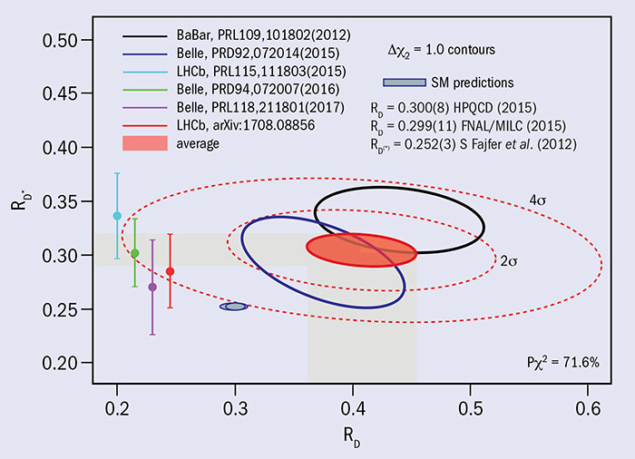Fig. 2. Status of RD and RD* measurements. The red ellipse represents the world average, including both the results from simultaneous measurements of RD and RD* (black and blue ellipses) and separate measurements of RD* (points with error bars). Image credit: HFLAV

In 2017, LHCb reported another RD* measurement by exploiting the decay of the τ lepton into three charged pions and a neutrino. This measurement was considered to be even more difficult than the previous one due to the large backgrounds from B decays and the apparent lack of discriminating variables. Nevertheless, the presence of a τ decay vertex significantly detached from the b-hadron decay vertex allows the most abundant backgrounds to be suppressed. The residual background, due to b-hadrons decaying to a D* and another charm meson that subsequently gives three pions in a detached vertex topology, is reduced by exploiting the different resonant structure of the three-pion system. The resulting measurement of RD* is larger than, although compatible with, the SM prediction, and consistent with previous determinations.

The combined world average of RD* and RD measurements, known to precisions of 5 and 10%, respectively, remains in tension with the SM prediction at a level of four standard deviations (figure 2). This provides solid motivation for further LU tests in semi-tauonic decays of B hadrons. In the next years, the LHCb collaboration will therefore extend the RD* measurement to the datasets collected in Run 2 and continue to study semi-tauonic decays of other b-quark hadrons.

In early 2018 the first measurement of RJ/ψ was performed, probing LU in the Bc sector. While the result was higher than the SM, the current uncertainty is large and the SM prediction is not yet firm. However, it can be an interesting test for the future. An important extension of this already rich physics programme, already being explored by Belle, will consider observables other than branching fractions, such as polarisation and angular distributions of the final-state particles. This will provide crucial insight when interpreting the current anomalies in terms of new-physics models.

### The plot thickens

The results described above concern tree-level semi-leptonic decays. In contrast, the other relevant class of transitions for testing LU, b s+, are highly suppressed because there are no tree-level FCNCs in the SM. This increases the sensitivity to the possible existence of new physics. The presence of new particles contributing to these processes could lead to a sizeable increase or decrease in the rate of particular decays, or change the angular distribution of the final-state particles. Tests of LU in these decays involve measurements of the ratio of branching fractions between muon and electron decay modes RK(*) = BF(B K(*)μ+μ)/BF(B K(*)e+e).

These modes represent a considerable challenge because the highly energetic LHC environment causes electrons to emit a large amount of bremsstrahlung radiation as they traverse the material of the LHCb detector. This effect complicates the analysis procedure, for example making it more difficult to separate the signal and backgrounds where one or more particles have not been reconstructed. Fortunately, there are several control samples in the data that can be used to study electron reconstruction effects, such as the resonant decays B K(*)(J/ψ e+e), and ultimately the precision is dominated by the statistical uncertainty of the decays involving electrons. Despite this, the LHCb measurements dominate the world precision.

Three measurements of RK(*) have been performed by the LHCb experiment with the Run 1 data: two in the B0 K*0+ decay mode (RK*) and one in the B+ K++ decay mode (RK). The results are more precise than those performed at previous experiments, and all have a tendency to sit below the SM predictions (figure 3). The BaBar and Belle experiments have also measured these LU ratios and found them to be consistent with the SM, albeit with a larger uncertainty.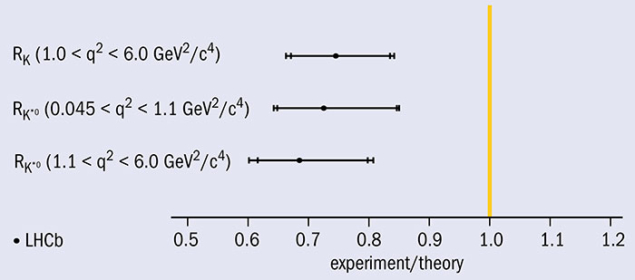Fig. 3. LHCb measurements of the lepton universality ratios RK and RK*. BaBar and Belle have made similar measurements, although with larger uncertainties.

Assuming that rather than being statistical fluctuations these deviations arise from new physics, one can ask the question: what is driving the RK and RK* anomalies? Is the electron decay rate being enhanced or the muon suppressed, or both? One could get an answer to this question by looking at the differential branching fractions of the decays B+ K+μ+μ, B0 K+0μ+μ and Bs0 → φμ+μ. Although with small statistical significance, all these branching fractions consistently sit below the SM predictions, indicating that something could be destructively interfering with the muonic decay amplitude. If a new particle was really contributing to the B decay amplitude, then one would naturally expect it to also influence the angular distribution of the decay products. Intriguingly, by studying the angular distribution of B0 K*0μ+μ decays one observes discrepancies that can be interpreted as being compatible with the expectation based on the central values of RK and RK*

Can we conclude it is due to new physics? Unfortunately not. Information such as branching fractions and angular observables are affected by non-perturbative QCD effects. In principle, these can be controlled, but there is an open question about whether the interference of fully hadronic decays such as B0 K*0J/ψ could mimic some of the discrepancies seen. This contribution is very hard to calculate and will most likely require controlling in the data directly.

All the results so far probing LU at LHCb are based on LHC Run 1 data recorded at a centre-of-mass energy of 7 and 8 TeV. Measurements of the RK and RK* ratios can be significantly improved over future years with the analysis of the full Run 2 data at an energy of 13 TeV. LHCb will also broaden its search for LU violation to other types of FCNC decays, such as Bs → φμ+μ. Another interesting avenue, recently taken up by Belle, is to compare the angular distributions of the decays B0 K*0μ+μ and B K*0e+e. If LU were indeed violated, then one would expect to see differences between the angular distributions of muons and electrons as well as the decay rates.

### Potential explanations

It is possible that the anomalies seen in tree-level and FCNC decays are related. The tree-level decays are sensitive to new physics at the TeV scale, whereas the FCNC decays are sensitive to scales of the order 10 TeV on account of the SM suppression of loop-level decays. If one would like to explain both anomalies with a single model, then this must also be suppressed in its contribution to b s+ decays compared to b cτ+ντ decays. This can be done by either forbidding FCNC processes at tree level, like in the SM, or by having a hierarchical flavour structure where the coupling to third-generation leptons is enhanced with respect to muons. Amongst several speculations, the most promising model in this regard introduces the well known concept of leptoquarks, which are particles that carry both lepton and quark quantum numbers (figure 4). The mass scale for such a leptoquark could be around 1 TeV, which is clearly very interesting for direct searches at the LHC.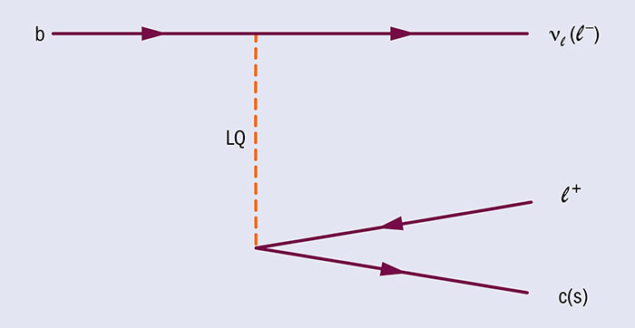Fig. 4. An example of a Feynman diagram for leptoquark-mediated b → cℓ+νℓ and b → sℓ+ℓ− singular transitions.

The theoretical options open up if one would like to explain only one set of anomalies. For example, the loop-level anomalies can be explained with a Z boson of a few TeV in mass, although the allowed parameter space for such a model competes with the constraints imposed by Bs matter–antimatter oscillations. Overall, there are many possible models proposed that can explain one or both of these anomalies, and differentiating between them would become an exciting challenge if these were to be confirmed.

In any case, the amount of data analysed for the measurements described here corresponds to just one-third of what will be available by the end of 2018 at LHCb. Meanwhile, following a major overhaul of the KEK accelerator, the Belle-II experiment is about to start operations in Japan and is expected to collect data until 2025 (CERN Courier September 2016 p32). The two experiments are designed for the study of heavy-flavour physics, and their complementary characteristics will allow researchers to perform ultra-precise measurements of decays of b-quark hadrons. Hence, the prospects for continuing to test lepton universality in the next decade and beyond are excellent.

BaBar Collaboration 2012 Phys. Rev. Lett. 109 101802.

BaBar Collaboration 2013 Phys. Rev. D. 88 072012.

Belle Collaboration 2015 Phys. Rev. D. 92 072014.

Belle Collaboration 2016 Phys. Rev. D. 94 072007.

LHCb Collaboration 2015 Phys. Rev. Lett. 115 111803.

LHCb Collaboration 2017 JHEP 08 055.

LHCb Collaboration 2014 Phys. Rev. Lett. 113 151601.

G Ciezarek et al. 2017 Nature 546 227.

F Archilli et al. 2017 Nature 546 221.

### Events

• Flavour physics | Conference FPCP 2024 27—31 May 2024 | Bangkok, Thailand
• Strong interactions | Conference SQM 2024 3—7 June 2024 | Strasbourg, France
• | Conference Neutrino 2024 16 June — 22 September 2023 | Milano, Italy